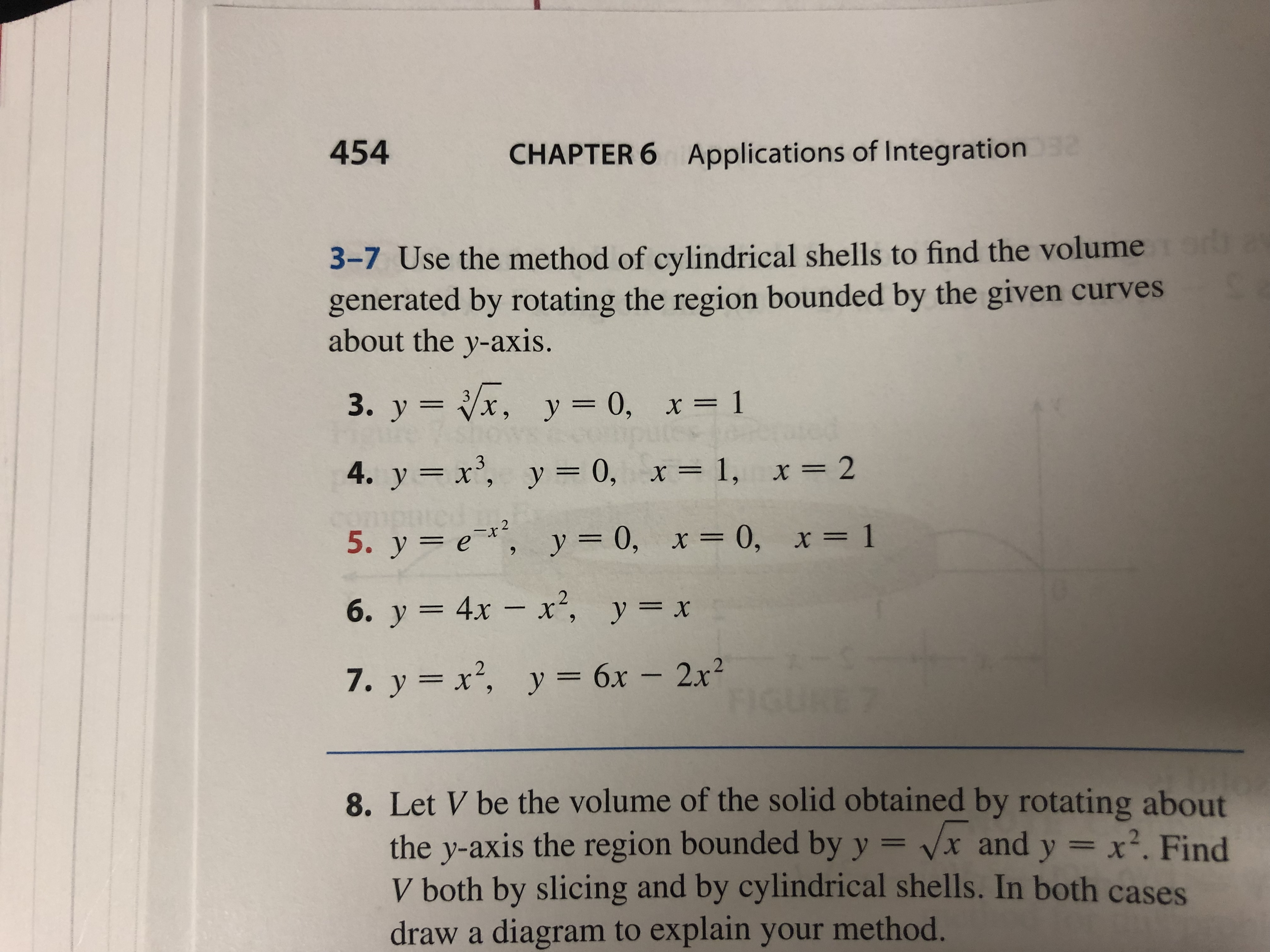# 454 CHAPTER 6 Applications of Integration 2 3-7 Use the method of cylindrical shells to find the volume generated by rotating the region bounded by the given curves about the y-axis. 3. y x, y = 0, x= 1 4. y x, y = 0, x= 1, x = 2 2 5. у %3D е*, у 3D0, х3D 0, х%3D 1 6. у 3 4х — х*, у%3D х 7. y x2, y 6x - 2x2 8. Let V be the volume of the solid obtained by rotating about the y-axis the region bounded by y = vx and y x2. Find V both by slicing and by cylindrical shells. In both cases draw a diagram to explain your method.

Question

#6help_outlineImage Transcriptionclose454 CHAPTER 6 Applications of Integration 2 3-7 Use the method of cylindrical shells to find the volume generated by rotating the region bounded by the given curves about the y-axis. 3. y x, y = 0, x= 1 4. y x, y = 0, x= 1, x = 2 2 5. у %3D е*, у 3D0, х3D 0, х%3D 1 6. у 3 4х — х*, у%3D х 7. y x2, y 6x - 2x2 8. Let V be the volume of the solid obtained by rotating about the y-axis the region bounded by y = vx and y x2. Find V both by slicing and by cylindrical shells. In both cases draw a diagram to explain your method. fullscreen

### Want to see this answer and more?

Experts are waiting 24/7 to provide step-by-step solutions in as fast as 30 minutes!*

*Response times vary by subject and question complexity. Median response time is 34 minutes and may be longer for new subjects.
Tagged in
MathCalculus

### Integration